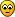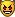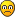## 17 clue puzzles

Everything about Sudoku that doesn't fit in one of the other sections

### 17 clue puzzles

Is there a link anyplace for the 17 clue collection?

I tried to find it, but it looks like the collection disappeared off the internet.

My computer died, and my new computer won't get here till Oct.

That being said. I think I might have that collection on one of my data drives.

BUT,

I'd still like to find it on the internet. Be way easier just to download it. Plus I wanted to try and install it on my phone.

Plus I'm not positive I ever did download the collection, in the first place.
Pupp

Posts: 246
Joined: 18 October 2019

### Re: 17 clue puzzles

Pupp wrote:Plus I'm not positive I ever did download the collection, in the first place.http://forum.enjoysudoku.com/17-clue-puzzle-collection-t38108.html
http://forum.enjoysudoku.com/17-clue-puzzle-difficulty-t38179.html1to9only

Posts: 3993
Joined: 04 April 2018

### Re: 17 clue puzzles

But, I must have the collection already.

If I can find it, I'll see if I can post it on the forum.

I'll break it up into 1000 puzzle chunks if I do
Pupp

Posts: 246
Joined: 18 October 2019

### Re: 17 clue puzzles

There are 49158 17-clues puzzles.
Don't think anyone will thank you for having to copy and paste from 50 posts.1to9only

Posts: 3993
Joined: 04 April 2018

### Re: 17 clue puzzles

I doubt anybody would thank me.

But then again, this forum seems to be a rare oasis.

I'll have to look at the file type, but maybe I can get Sudocue to host the file. But as a last resort, posting the puzzle in 50 sections would at least keep it on the internet.

No idea how many were computer generated, but no doubt many people hand crafted some of them. It would be a shame for all that effort, over many years, if not decades, to be lost forever from the internet.

Posting isn't my first choice, though.

Sometimes archiving data is a thankless job.Pupp

Posts: 246
Joined: 18 October 2019

### Re: 17 clue puzzles

Pupp wrote:It would be a shame for all that effort, over many years, if not decades, to be lost forever from the internet.

I'm pretty sure a rummage through the internet will dig up a few 17-clues puzzles lists.
1to9only

Posts: 3993
Joined: 04 April 2018

### Re: 17 clue puzzles

Here from my goggle drive , any would be pretty difficult to hand craft, but they will probably never be lost, but perhaps somewhere in another universe someone will discover them all again ...

17 clue puzzles
coloin

Posts: 2175
Joined: 05 May 2005
Location: Tenerife

### Re: 17 clue puzzles

You can also find the 49158 17-clue puzzes as one of the test datasets in tdoku project on github.
Specifically the file "puzzles2_17_clue" found within data.zip
tdillon

Posts: 60
Joined: 14 June 2019

### Re: 17 clue puzzles

Here is a list of 8.4 and higher :
Code: Select all
`..............1.23..456...............7.8.4...1...25.....6......2.....1.3...4.... ED=9.1/1.5/1.5..............1..2.34....5.......3......67....5....48....8.....12......76..4..... ED=9.0/1.2/1.2..............1..2.34....5.......3......67....5....48....8.....21......76..4..... ED=9.0/1.2/1.2..............1.23.45.6...1........7....4.8..2..9........2...9...4........7...5.. ED=8.9/1.2/1.2..............1.23.45.6...1........7....4.8..2..9........2...9...6........7...5.. ED=8.9/1.5/1.5..............1.23.45.6...1........7....4.8..2..9........2...9...6.......7....5.. ED=8.9/1.5/1.5........1.......2...3..4.........3.5.....64...7..2.......17..8...4.8....6.5...... ED=8.9/1.2/1.2................12..3..4.........3...1..5....65.7....4...81..5...2....9...4...... ED=8.9/1.2/1.2........1.......23..4..5.........4.5.3..16...7............3..8...51.....6.8...... ED=8.8/1.2/1.2........1.......23..4..5........65...2........3...1.......3...7..64..8..5..9..... ED=8.8/1.2/1.2........1.....2.3...4..5...........6....4.7...52..3.........35.8........9..16.... ED=8.5/1.2/1.2...............123..4..5........4..5.1...6...62.....7.....2......8...4...7.3..... ED=8.5/1.2/1.2..............1..2..3.4..5.........6..735.....8......1...7.2.3..1.6.....4........ ED=8.5/1.2/1.2..............1..2.34....5........3.....26.....5...47....7.....1..4.....68......1 ED=8.5/1.2/1.2..............1..2.34....5........3.....26.....5...47....7.....16......82..4..... ED=8.5/1.2/1.2..............1..2.34....5........3.....26....5....47....7.....1..4.....68......1 ED=8.5/1.2/1.2..............1..2.34....5........3.....62....5....47....7.....21......86..4..... ED=8.5/1.2/1.2..............1..2.34....5........3.....67....5....48....8.....12......77..4..... ED=8.5/1.2/1.2..............1..2.34....5........3.....62....5....47....7.....21......86..4..... ED=8.5/1.2/1.2..............1..2.34....5........3.....67....5....48....8.....12......77..4..... ED=8.5/1.2/1.2..............1..2.34....5......2.......3.5....6....4....75..3.12......68........ ED=8.5/1.2/1.2..............1..2.34.5..6.......53...7...4..2....8......34.7.....7.....1........ ED=8.5/1.2/1.2........1.......2...3.45.........4.6..76......1.2.8......1.......4...5..9...3.... ED=8.5/1.2/1.2........1.......2...3.45........6..3.2.7.....4.....5.....1........36....8.5....7. ED=8.5/1.2/1.2........1.....2.3...4..5...........6....4.7...52..3.........35.8........9..16.... ED=8.5/1.2/1.2..............1..2..3..4.5.....1........2..3..65...4.....3......7...6..12.......8 ED=8.4/1.2/1.2..............1..2..3.4..5.........6..7.3.....8...2..1.......4....75..3.16....... ED=8.4/1.5/1.5..............1..2..3.4..5......2..1..4.....6..5.3........7..4..3.......62...8... ED=8.4/1.2/1.2..............1..2.34....5........6.....72.....5...43....3.......64.....7...1...8 ED=8.4/1.2/1.2..............1.23.45.6...1........6....4.7..2..8........2...8...4.......6....5.. ED=8.4/1.2/1.2........1.......2...3.45.........3...1.......26...7........246...8....7.1..9..... ED=8.4/1.2/1.2................12..3..4..5.....5....1.....67.8...9.......1..8...5...9..4...6.... ED=8.4/1.5/1.5................12..3.45.........3.....1.6..745...2.......5......8.9.7..2........ ED=8.4/1.2/1.2........1........2..3..4........5.6..1.....4.72...........1.8.7...92.....36...... ED=8.4/1.2/1.2........1.......2...3..4..5.....6....7..3.4..21.....8.....1.......25......9...7.. ED=6.7/6.7/2.6..............1..2.34....5.......3......5..4.12...6......2.......5.3..7.8.......6 ED=7.2/3.4/2.6`

There are no tough pearls. The last two listed are the closest.
There are 56 puzzles with 8.3. Six puzzles with 8.2. Then there are six puzzles with 7.9.
7.8 42
7.7 37
7.6 48
7.4 2
7.3 79
7.2 478
7.1 1023
7.0 14
ghfick

Posts: 159
Joined: 06 April 2016

### Re: 17 clue puzzles

Here are the 8.3 puzzles down to the 7.3 puzzles :
Code: Select all
`.................1..2.34.................526..7.8........98...7..6....3.2.51..... ED=8.3/1.2/1.2................12....34.........3....1....5..675........27...834......69........ ED=8.3/1.2/1.2................12....34.........3....5.....1.671........27..8.34.....6.9........ ED=8.3/1.2/1.2................12....34.........3....5.....6.786........28..7.34.....2.9........ ED=8.3/1.2/1.2................12....34.........3....5...4.6.7.2.........6.5.3.8.......12.7..... ED=8.3/1.2/1.2................12..3..4.................34.516.....2.....17.8....86.3....5...... ED=8.3/1.5/1.5................12..3..4...........3.2.56....7.....4.8....2..5....19......8...3.. ED=8.3/1.2/1.2................12..3..4...........3.2.56....7.....4.8....2..5....91......8...3.. ED=8.3/1.2/1.2................12..3..4........1....5......672......5....8..3...1...4...7.2...9. ED=8.3/1.2/1.2................12..3.45..............6..14...7.2.8.......7......9.6.3...1......8 ED=8.3/1.5/1.5................12..3.45.........4...16......72.3........2...7...4...5...6...8... ED=8.3/1.2/1.2................12..3.45........6......1...7...5...4......8.5...2......671.9..... ED=8.3/1.2/1.2................12..3.45......1...67..4.......5...2......78.4...1.......7..2..... ED=8.3/1.2/1.2...............123..4.56...........7.5.....6.18.2..........7......1..8....6....5. ED=8.3/1.5/1.5..............1..2..3....4.........5....4..3..2...61.7....3..8..7.......21...7... ED=8.3/1.2/1.2..............1..2..3....4.........5..4.3.....2...5..1..6.7..3..1.....8..5...6... ED=8.3/1.2/1.2..............1..2..3....4.........5..6...1....789.....3..4..9..5...7...21....... ED=8.3/1.2/1.2..............1..2..3....4......53......1..6.78...9......34......96......2......8 ED=8.3/1.2/1.2..............1..2..3....4......567..1.......4.2..8.......6.1......9.8....74..... ED=8.3/1.5/1.5..............1..2..3..4.5......3....16...7..7.......8....6.3......9.4..2..7..... ED=8.3/1.2/1.2..............1..2..3.4..5.........6.....21.7.453.......895....1........7........ ED=8.3/1.2/1.2..............1..2..3.4..5.........6.....21.7.458.......835....1........7........ ED=8.3/1.2/1.2..............1..2..3.4..5.......34..1...6...2......7......2..8..4.5......5.....6 ED=8.3/1.2/1.2..............1..2..3.4..5.......46..2........78.........2.7..1...9.....5.4....8. ED=8.3/1.2/1.2..............1..2.34....5..............5.34.1..6.2...........7..5.4....2..8....1 ED=8.3/1.5/1.5..............1..2.34....5............5.3.64.1.7..2......4.....2.......18...5.... ED=8.3/1.5/1.5..............1..2.34....5............6...73.1...28......3...4....7....88.......9 ED=8.3/1.2/1.2..............1..2.34....5.........6..5...43.7...12.......5...8...3.....2.......1 ED=8.3/1.2/1.2..............1..2.34....5........6......534.2.7..........4......536....8.......1 ED=8.3/1.2/1.2..............1..2.34....5........6......734.2.5..........4.....5.36....8.......1 ED=8.3/1.2/1.2..............1..2.34....5........6...5.4....2..7....1......3......5..4.1..8.2... ED=8.3/1.5/1.5..............1..2.34....5.......6.7..546......8...1.....9...3.1....2...7........ ED=8.3/1.2/1.2..............1..2.34....5.......63.1....2...6......7.....5.......43....2....6..8 ED=8.3/1.2/1.2..............1..2.34....5.....3........6....21......7...3.......5...46.7..2.8... ED=8.3/1.2/1.2..............1..2345....6.......34.....7......2..8.5.....4.......36....7.......1 ED=8.3/1.2/1.2..............1.23..4.5...6............74.5..23......1........8..7...4...1...3... ED=8.3/1.5/1.5..............1.23..4.5...6....4.7...1.......3..7........2..8....5...1...6...3... ED=8.3/1.5/1.5..............1.23..456............7....23.6..18.........1........4.81..2........ ED=8.3/1.2/1.2..............1.23.45.6............7..8...5..2....3......8.......7.5.4..1......3. ED=8.3/1.2/1.2..............1.23.45.6...1........6....7.8..2..9........2...9...6...4...7....... ED=8.3/1.5/1.5...........1..2..3..4..5.6.......7.1.3.......5............8.......14...765.....3. ED=8.3/1.2/1.2........1........2..3..4..........3....15......67...5......378..9.......12..6.... ED=8.3/1.2/1.2........1.......2...3..4........5.......364..17..........8..3...56......2..1....8 ED=8.3/1.2/1.2........1.......2...3..4........56...1......37............5.3..62....4..8..12.... ED=8.3/1.2/1.2........1.......2...3.45.........6.....1....3..7....8......74...2...8...14....3.. ED=8.3/1.2/1.2........1.......2...3.45........63...1.......72..5.......2....7..1...6...8....5.. ED=8.3/1.2/1.2........1.......23....45........264...7.......318........1......8.......4....6.9. ED=8.3/1.2/1.2........1.......23....45........64....2.......31........723.....5..8....4.....6.. ED=8.3/1.2/1.2........1.......23....45........674...8.......319........1.......9......4....7.6. ED=8.3/1.2/1.2........1.......23..2..4.......1.......3....5.64...7.....8...6..5...64..3........ ED=8.3/1.2/1.2........1.......23..4..5.........46.....2.....1..3........1......67.....4.58..6.. ED=8.3/1.2/1.2........1......23...4.56..........5..2.....6..782........3..7....1......5....4... ED=8.3/1.2/1.2........1.....2.....3....45......26....34.....5....7.....1....8.2...6...47....... ED=8.3/1.2/1.2........1.....2....34.....5........3....6..7..5.8........5.......2...76.7..4...2. ED=8.3/1.2/1.2........1.....2..3..4.56.........26..7.......81.3........17......6....5..4....... ED=8.3/1.2/1.2........1.....2.3...4.5........6.4...2.......3....1.7....7.......6...5.48....3... ED=8.3/1.2/1.2................12..3.45...............1....6.74.3........8.5..19.2.....6.....4.. ED=8.2/1.5/1.5................12..3.45.........4...1..6....72.8........2....7..4...5...6..9.... ED=8.2/1.2/1.2..............1..2..3...45............456.....7......1........8.....21.75.6.3.... ED=8.2/1.2/1.2..............1..2..3...45............647.....8......1........9.....2.184.7.3.... ED=8.2/1.2/1.2..............1..2..3.4..5......25....6...43..7...8.......3..6..1.......2.......8 ED=8.2/1.2/1.2..............1..2.34....5.......34...5....6.7....2.......4....2...6...18..5..... ED=8.2/1.2/1.2.................1..2.34........2....4..5....6..1....7.....8.3...3...54.1..6..... ED=7.9/1.2/1.2................12..3..4..............5.6.3.4.2.71.........54...7....8..81....... ED=7.9/1.2/1.2................12..3.45...........6...2...7..45..1.......3.5..2.....1..7..8..... ED=7.9/1.2/1.2..............1.23..456..........5....7...4...1..82......6......2.....1.3...4.... ED=7.9/1.5/1.5..............1.23.45.6...7....4.5....1......3...........8.3....5....6...8...2... ED=7.9/1.2/1.2........1......23...4.56..........74.....3....1.2.........4..8..2..7....36....... ED=7.9/1.2/1.2.................1..2..3.4......5.2..6...7...81...........1.3.6....8......96..2.. ED=7.8/1.2/1.2................12....345........3....67......581..........54..2.1....7.3........ ED=7.8/1.2/1.2................12..3..4...........3..4.5.6...7..1.......8.64...2.......51....8.. ED=7.8/1.2/1.2................12..3..4..5.....36...2...7...4......8..1.28.....8.......9.......7 ED=7.8/1.2/1.2................12..3.45...........6.....3....7.2....4.....6.3.4.....5..82.1..... ED=7.8/1.2/1.2................12..3.45.........4...14....3..6...2.......3........7..8.52......6 ED=7.8/1.2/1.2................12..3.45........3......1....6.75...8.......75...1.2.....6......3. ED=7.8/1.5/1.5................12..3.45........6..7..4....26.2.5........1.....6.....5..8...2.... ED=7.8/1.2/1.2................12..3.45........63...1.......7.......8...2....6..4.1....3.6...5.. ED=7.8/1.2/1.2................12..3.45........63...1.......7......8....2...6...4.1....3.6...5.. ED=7.8/1.2/1.2................12..3.45........65...7.......61.2...8....1.......5...4...3...7... ED=7.8/1.2/1.2................12..3.45.......134...2.6.....7...........7...28..4......5.......7 ED=7.8/1.2/1.2................12..3.45......1...6..4....5..13..........3........7.6...28....4.. ED=7.8/1.2/1.2...............123..4..5................1.6...23.....5.....3..4.1..7.8..6....4... ED=7.8/1.2/1.2..............1..2..3..4.5.....6.1....5.3.....7....8.....25..3..8.......1.....7.. ED=7.8/1.5/1.5..............1..2..3..4.5.....6.1....53......7....8.....25..3..8.......1.....7.. ED=7.8/1.5/1.5..............1..2..3.4..5.........6.....2..1.45..........5..4.1...37...2.......8 ED=7.8/1.5/1.5..............1..2..3.4..5.........6..523.....7.....81....5..3.1........6....2... ED=7.8/1.2/1.2..............1..2..3.4..5.........6..523.....7.....81....9..3.1........6....2... ED=7.8/1.2/1.2..............1..2..3.4..5.........6..7.....1..859...3....5..4..6.......21....... ED=7.8/1.2/1.2..............1..2..3.4..5.........6..7.....1..875........9.4...6......321....... ED=7.8/1.5/1.5..............1..2..3.4..5........4..1...6...62..........7.8..6..5......3.4.....9 ED=7.8/1.2/1.2..............1..2..3.4..5........4..2...6...61..........7.8..6..5......3.4.....9 ED=7.8/1.2/1.2..............1..2..3.4..5.......1.6..7.8.9...5..........37.....2..5....1.....6.. ED=7.8/1.2/1.2..............1..2.34....5.........6..745.3..2.......1......74.1....8...6........ ED=7.8/1.5/1.5..............1..2.34....5.......6....235......7.....1.1.4......6.......8..5...3. ED=7.8/1.2/1.2..............1.23..4.56.........6...2.47....7..8........2....7..6...5...3....... ED=7.8/1.2/1.2..............1.23.45.6............4..3...5..2....7..........7...43......7...8.1. ED=7.8/1.2/1.2........1.......2...3.45........34.5.2.6.....7...........2....8..4.....36..7..... ED=7.8/1.5/1.5........1.......2...3.45........46.3.7.......12..........1........73.4...8.....5. ED=7.8/1.2/1.2........1.......2...3.45........46.3.7.......21..........1........73.4...8.....5. ED=7.8/1.2/1.2........1.......23..4..5.........4....2..6.7..8..3.........2....3......81..6.4... ED=7.8/1.2/1.2........1.......23..4..5.........4....5.6.7...3.1..........68.7.1.......2..8..... ED=7.8/1.2/1.2........1.......23..4..5........24...2...6...37...........3.......17..8...9...2.. ED=7.8/1.2/1.2........1.......23..4..5........46......78...12..........3...1...7......6....98.. ED=7.8/1.2/1.2........1.......23..4..5........6.......7.4..23.....8....38......7...9..5...2.... ED=7.8/1.2/1.2........1.......23..4.56.........4.7.....3....1.2.........4...8.3.......26..7.... ED=7.8/1.2/1.2........1.......23..4.56.........4.7.....3....1.2.........4.8...3.......26..7.... ED=7.8/1.2/1.2........1.......23..4.56........2..7..6...1...1.3.........8.5...2.......7...9.... ED=7.8/1.2/1.2........1.....2.....3.4..5......6..7..4.....2.8.7........1..43.2...7....6........ ED=7.8/1.5/1.5........1.....2.....3.4..56........3.2.6.....4.....7.......18.......42...16...... ED=7.8/1.2/1.2........1.....2.3...4.5........6.7...2.1.....3.....8.....3.......7..8....18.....6 ED=7.8/1.2/1.2................12....34........53....1.......261..7.....2....5.7..8....3.....4.. ED=7.7/1.2/1.2................12..3.45.............1.6.....27.1...8.......4....4.7.5...6.2..... ED=7.7/1.2/1.2................12..3.45.........4....56..3...4..1.........37...8.......12.....8. ED=7.7/1.2/1.2................12..3.45........36.4.1...4....7..........1..5....6...3...8.7..... ED=7.7/1.2/1.2................12..3.45........6.....4...5...7.1......1.2......8.......5....346. ED=7.7/1.5/1.5................12..3.45........65.4.1.......7...8.......1...7...9...8...4...2... ED=7.7/1.5/1.5...............123..4..5...........4.3...1...16..2..........3.5....3......76....8 ED=7.7/1.2/1.2..............1..2..3..4.5.....3......67....8.4......1....8..6.1....2...7......3. ED=7.7/1.5/1.5..............1..2..3.4..5.........6..7.....1..853........5.4...6.......21.....3. ED=7.7/1.2/1.2..............1..2..3.4..5........4..2.......61...2......7.8..6..5......3.4.....9 ED=7.7/1.2/1.2..............1..2..3.4..5.......4........63..7...2......5......8....1..4.563.... ED=7.7/1.2/1.2..............1..2..3.4..5.......6....253.....7......1....8..3..17...4...6....... ED=7.7/1.5/1.5..............1..2..3.4..5.......6....753.....2......1....8..3..1...24...6....... ED=7.7/1.5/1.5..............1..2.34....5.....5..3.1....2...67....8.....8.....2.......17...3.... ED=7.7/1.5/1.5..............1..2.34.5..6........3..57...4..2..8...........2.....63....1.......8 ED=7.7/1.2/1.2..............1..2.34.5..6.......2.....63....7.......8......5.....97..3.2....8... ED=7.7/1.2/1.2..............1..2.34.5..6.......35....6.....1....2.......3...72.......18..4..... ED=7.7/1.5/1.5..............1.23..4.5...6....4.5...2.......7...........7..86...5...4...1...2... ED=7.7/1.5/1.5..............1.23..4.56.........6...2.78....8..4........2....8..6...5...3....... ED=7.7/1.2/1.2..............1.23.14.5............6.2.....4.7..3.8.......4..1...6......3.......8 ED=7.7/1.5/1.5..............1.23.45..6...........7..7...4..2...3.......4..5...8.....6.3..7..... ED=7.7/1.2/1.2...........1..2..3.4..5..6.........13...7....6.....5.....1..4....3..8...5......7. ED=7.7/1.2/1.2........1........2..3.45.6......35...1.......72...........8.......12...7..6...3.. ED=7.7/1.2/1.2........1.......2...3..4.........3....4...5...1..26.7....2.....3..8.....6.5...4.. ED=7.7/1.2/1.2........1.......2...3..4.........3....4.5.....6.71.........34...1......62..5.8... ED=7.7/1.2/1.2........1.......2...3..4.........3.5..1.2.....6.7..........1.8.32....4..7...5.... ED=7.7/1.2/1.2........1.......2...3..4........1.......3.5..26.....7....2.......57..4...48.....3 ED=7.7/1.2/1.2........1.......2...3.45.........4....56..3...4..1.........37...8.......12......8 ED=7.7/1.2/1.2........1.......2...3.45........3.....6.......1.2....7...4..3...7....5..82..6.... ED=7.7/1.2/1.2........1.......2...3.45........34.......6...12......7..8...5..5.....6..7..1..... ED=7.7/1.2/1.2........1.......2...3.45........35...1..6.....7.2........7....8.2..1....5.....3.. ED=7.7/1.5/1.5........1.......2...3.45........63...1.2.....7.......8...1...7...5...6...6...8... ED=7.7/1.2/1.2........1.......2...3.45........64...7.......12.8........7...1...4.....6..5...3.. ED=7.7/1.2/1.2........1.......23.....4........56.7..2........3.7.....1.3.....5.....4..7....68.. ED=7.7/1.2/1.2........1.......23..4..5........67......48....1......5...19......6...8..7.....4.. ED=7.7/1.2/1.2........1.....1..2..3.4...........5...67..3...2...8.......6.4...1......85..3..... ED=7.7/1.2/1.2........1.....2.....3....45.......5..6...72..8...46.......3......45......2....7.. ED=7.7/1.2/1.2................12..3..4.........5.6.....3..728..4.......2...4....81......7...3.. ED=7.6/1.2/1.2................12..3..4.........5.6.....3..728..9.......2...9....81......7...3.. ED=7.6/1.2/1.2................12..3..4........13.5.2......4.6..7........5......4......8..62..7. ED=7.6/1.2/1.2................12..3..4........3....1.......52..6..7....2....3..8...4...7.5.1... ED=7.6/1.2/1.2................12..3..4........34...5.......12......6....7..5...8...3..6..52.... ED=7.6/1.2/1.2................12..3..4........54...1........62..........1..7.....2.3..4...685.. ED=7.6/1.2/1.2................12..3..4..5.....6.......21..7.58...3.....8......6.4.....1......6. ED=7.6/1.2/1.2................12..3.45...............1.2..6.54..........7.4..128....3.6........ ED=7.6/1.2/1.2................12..3.45...............1.6..7..48..3...2.......6...9.4..71....... ED=7.6/1.2/1.2................12..3.45..............4.63....1.....27......5.....7..3..87.1..... ED=7.6/1.2/1.2................12..3.45.............6.1.....12.7...8.......4....4.6.5...7.2..... ED=7.6/1.2/1.2................12..3.45.............6.1.....21.7...8.......4....4.6.5...7.2..... ED=7.6/1.2/1.2................12..3.45...........6..4..3....2.7........1..34...7...5...8.2..... ED=7.6/1.2/1.2................12..3.45.........3...1.......6.7..45.....21..8....8.....9.5...... ED=7.6/1.2/1.2................12..3.45.........3.6......45..7.1.......5.......1.7.....8.62..... ED=7.6/1.2/1.2................12..3.45.........4...1.......62.3.........78......2....6..4..65.. ED=7.6/1.2/1.2................12..3.45........1.....6...7....8.5..9.....7.5...1.3.....29....... ED=7.6/1.2/1.2................12..3.45........65....7...4...8.1.......4.......1.2...8.5.....7.. ED=7.6/1.5/1.5................12..3.45......1.6.3...4...7...2..........8..5.43...1....6........ ED=7.6/1.2/1.2..............1..2..3..4.5...........1.26....7.....43....5.3....2......68.....1.. ED=7.6/1.5/1.5..............1..2..3..4.5........6...7.3.....8.9....1....5.83..9.......21....... ED=7.6/1.2/1.2..............1..2..3..4.5.......31..2..6....7............7.......62..8.8.1....9. ED=7.6/1.2/1.2..............1..2..3..4.5.....2...1.3....6...7..........5.74....1......6.2....8. ED=7.6/1.2/1.2..............1..2..3.4..5........36.......4..1...7......834....2....1.76........ ED=7.6/1.2/1.2..............1..2.34....5........3.....2.6..2.1..7.......3..4..8.5.....1.......7 ED=7.6/1.2/1.2..............1..2.34....5........3..6....4..1....2.......3........5...16.74....8 ED=7.6/1.5/1.5..............1..2.34....5........3.1....2...6.....74.....5.......43...82.7...... ED=7.6/1.2/1.2..............1..2.34....5.......3..1...62...7.....8......8.......3..4..2.5.....1 ED=7.6/1.5/1.5..............1..2.34....5.......34......6...16...7.......3....2.....7.16..8..... ED=7.6/1.2/1.2..............1.23..4.5...6......5......4..6.13...7........3......1.2.....6...8.. ED=7.6/1.2/1.2..............1.23.45.6..........4......5.......2.3..7....8.5...6.....4.3....7... ED=7.6/1.2/1.2...........1..2..3.4..5..6.......1....5...2..7...6.........14..5........6..3...8. ED=7.6/1.5/1.5........1.......2...3..4.........5.3.2.......6.7..84......5.......21..5...4...9.. ED=7.6/1.2/1.2........1.......2...3.14.........4.3...5......6.2..........37...5...1...82.....5. ED=7.6/1.2/1.2........1.......2...3.45.........3.4.6.......12..7......5.......8.6.....7..2...1. ED=7.6/1.2/1.2........1.......2...3.45......1.2..6...3..5....4.......1.......27.......6...8.4.. ED=7.6/1.2/1.2........1.......2..34.56...........3..5...1..7.82..........9.8.....3....1.......2 ED=7.6/1.2/1.2........1.......23....45........64....2.......73.........21..7..5....8..4..3..... ED=7.6/1.2/1.2........1.......23....45........64....3.......72.........21..7..5....8..4..3..... ED=7.6/1.2/1.2........1.......23..4..5.........4.......2.5.16..3........1...7.1.6..8..2........ ED=7.6/1.2/1.2........1.......23..4..5.........4...1..3.....6..7......5...8...3..6....2..31.... ED=7.6/1.2/1.2........1.......23..4..5.........46...5.4.7...1..........21......73.....5.....8.. ED=7.6/1.2/1.2........1.......23..4..5........6..7..8...6...2..1........7.......23..1.9.5...... ED=7.6/1.2/1.2........1.......23..4..5........65...3..2....7.......8....1.......3...7.6.5...8.. ED=7.6/1.2/1.2........1.....2.....3....45......6......3...7.1.4.........8..9..2...1...4.7...2.. ED=7.6/1.2/1.2........1.....2..3..4.5...........6...2...4...3...1.......2.....1.....788..64.... ED=7.6/1.2/1.2........1.....2.3...4.5........6.4...2.....7.3....8......9......46...5...8...3... ED=7.6/1.2/1.2........1.....2.3...4.5........6.4...2.....7.3....8......9......46...5..8....3... ED=7.6/1.2/1.2..............1..2..3.4..5.........1..6....3..7...8.......6.....9....8.74..53.... ED=7.4/1.2/1.2..............1..2.34....5........1.......3..6.7..8......35......8.4....7..9....6 ED=7.4/1.2/1.2.................1..2.34........2.3..1...5....6.7..8.....6.......4....2..8.1..7.. ED=7.3/1.5/1.5................12....34........53....1....6..721........26...8.9.......34....... ED=7.3/1.2/1.2................12..3..4.........3.5.1...2....6..4........6......517......8...52. ED=7.3/1.2/1.2................12..3..4.........3.5.1...2....6..4........65.....517......8....2. ED=7.3/1.2/1.2................12..3..4.........3.5.2..1.....6..7.......89...3..4...5...7.2..... ED=7.3/1.2/1.2................12..3..4........35...6.......12......7....7.3.....82......91..4.. ED=7.3/1.2/1.2................12..3..4........53...1.....6..7..3.......18..7...46......25...... ED=7.3/1.2/1.2................12..3..4........54...1.....6..7...3.......1.7....58..3..8...6.... ED=7.3/1.5/1.5................12..3.45........1.5...6...7...8.2.........6.38..1.......7...9.... ED=7.3/1.5/1.5................12..3.45........3..6.1.6.....4.....5......57.4...6...8...2....... ED=7.3/1.2/1.2................12..3.45........43...1.62..7.8............7.......1...8...4...5.. ED=7.3/1.2/1.2................12..3.45........63...4....5...7.1........21..7...5.......8.7..... ED=7.3/1.2/1.2................12..3.45........63...4....7.58..9........2........81.....25...... ED=7.3/1.2/1.2..............1..2..3....4.............34..5.16......7...58..3.2....6..17........ ED=7.3/1.5/1.5..............1..2..3....4.........1..536.....2.7....8.......5...69...3..9...8... ED=7.3/1.2/1.2..............1..2..3....4.........5..637.....2......1....6.87....4...3.59....... ED=7.3/1.2/1.2..............1..2..3....4......5..1..6.3.....4......7....8..3.1..4.7...5......6. ED=7.3/1.5/1.5..............1..2..3...45..............4.36718...2......35......7.......2......8 ED=7.3/1.5/1.5..............1..2..3..4.5..............6.73..1...8..4...........653....2.....1.8 ED=7.3/1.5/1.5..............1..2..3.4..5..............3..6..7...2..8.......4.....6.53.92...7... ED=7.3/1.2/1.2..............1..2..3.4..5.............2..6.1.54....3.....3..472..8.....6........ ED=7.3/1.5/1.5..............1..2..3.4..5........6..1...7...3.5....4.......8.1..69......2......7 ED=7.3/1.2/1.2..............1..2..3.4.56.....3.......5......7......8...7....1..5..8...6.4....3. ED=7.3/1.2/1.2..............1..2.34.....5....3..6...1...7..2...5.......6.71...5......86........ ED=7.3/1.2/1.2..............1..2.34....5............635....2...4.7.1.......3..8......91....7... ED=7.3/1.5/1.5..............1..2.34....5........3.....6....71......8...4.......653....2.....7.1 ED=7.3/1.2/1.2..............1..2.34....5........3.1........6....71......5...8...43....2.8.....6 ED=7.3/1.2/1.2..............1..2.34....5........3.16.......2..57..........6.....34....68......9 ED=7.3/1.2/1.2..............1..2.34....5........6......2.....5...74.....4..7.1.6.....82..7..... ED=7.3/1.2/1.2..............1..2.34....5.......34.....6....2....7.......4......1.56..78.......9 ED=7.3/1.5/1.5..............1..2.34....5.......6.....35....2.......1...6...3...7...4..1...82... ED=7.3/1.5/1.5..............1..2.34....5.....3.1....5.6....7....2..8...7...3.18.......2........ ED=7.3/1.5/1.5..............1..2.34.5..6........3.......5..7....8........27...5......8.693..... ED=7.3/1.2/1.2..............1.23....45..6.....74....8.......32.........2...8.5.....7..7..3..... ED=7.3/1.2/1.2..............1.23..4..5..6......71.....8......39........3....9.5.......17.....5. ED=7.3/1.2/1.2..............1.23..456..........5.6.1.......27............3......4.2.7...6.....8 ED=7.3/1.2/1.2..............1.23.45.6............1...64....2......7.......4.....2.7...1.8...5.. ED=7.3/1.5/1.5..............1.23.45.6............7..65.....2....3.........4........56839....... ED=7.3/1.2/1.2...........1..2..3.4..5..6.........7......1.2.8.4.........6..8...7......2.3..1... ED=7.3/1.2/1.2........1........2..3..4..........5....12..6...7.8.....2....7..18.......6....54.. ED=7.3/1.2/1.2........1........2..3..4........345..1.......6....78......5.3...9.26....2........ ED=7.3/1.2/1.2........1.......2.....34......1...5...6...7....85......5........9...63..21...3... ED=7.3/1.2/1.2........1.......2.....34......1...5...6...7....85......5........9...64..21...3... ED=7.3/1.2/1.2........1.......2...3..4........34.5..6......12...........6.7...8....3..5..71.... ED=7.3/1.2/1.2........1.......2...3..4........35.6.21......7...........26...8..9...4...5.7..... ED=7.3/1.2/1.2........1.......2...3..4........5..6..7...4...2..1...5...7.83...6....9..1........ ED=7.3/1.5/1.5........1.......2...3..4........53...1..2....6.7.....8...1........6.39...5....4.. ED=7.3/1.5/1.5........1.......2...3..4........54.6.7.......21...........7.8....69..3...9..2.... ED=7.3/1.2/1.2........1.......2...3..4........56.3.2...3...7............2.4....58..3...9..7.... ED=7.3/1.2/1.2........1.......2...3.45.........4....6.735...1..........21...8...8.....9.5...... ED=7.3/1.2/1.2........1.......2...3.45.........4.6....3.......27..1......63.8.7.......12....... ED=7.3/1.2/1.2........1.......2...3.45.........4.6....3.......27..1......63.8.7.......21....... ED=7.3/1.2/1.2........1.......2...3.45........4........65...17....8....21......6.7.3..5........ ED=7.3/1.2/1.2........1.......2...3.45........6....1.7.....2.8....4....9.1..7..4...9...2....... ED=7.3/1.2/1.2........1.......23....45........6.....3...7...21.........3...1.5...894..6........ ED=7.3/1.2/1.2........1.......23..4..5..........6......75..12..3.......12...8...4......76...... ED=7.3/1.2/1.2........1.......23..4..5..........6.....3..7..23.1..........4...1.7.....7....6.8. ED=7.3/1.2/1.2........1.......23..4..5.........6......784...3.1........23...9..5......6.7...... ED=7.3/1.2/1.2........1.......23..4..5........46...1........2..7........3.......21.8..7.56..... ED=7.3/1.2/1.2........1.......23..4..5........46...1........2..7........3.......21.8..9.56..... ED=7.3/1.2/1.2........1.......23..4..5........46...1..7.....2...........3.......21..7.8.56..... ED=7.3/1.2/1.2........1.......23..4..5........46...1..7.....2...........3.......21.8..7.56..... ED=7.3/1.2/1.2........1.......23..4..5........46...1..7.....2...........3.......21.8..9.56..... ED=7.3/1.2/1.2........1.......23..4..5........65...3..1.....7...........3...2..6.8....9.52..... ED=7.3/1.2/1.2........1.......23..4..5........67...1........2..4........3.......21.8..9.57..... ED=7.3/1.2/1.2........1.......23..4..5........67...1..4.....2...........3.......21.8..9.57..... ED=7.3/1.2/1.2........1.......23..4..5.......1......67..8...3......5....2.7..1....3...8.....9.. ED=7.3/1.5/1.5........1.......23..4.56........5.....7.......1.2....8....7.....2.8.....64....5.. ED=7.3/1.2/1.2........1.......23..4.56........76...3.2......8..........1....8..7......5.6..4... ED=7.3/1.2/1.2........1......234..5..6........7.8..1..5.....3..........1..6.....4.....6.7....2. ED=7.3/1.2/1.2........1.....1..2..3.4..........35...6.......2...7.......6.8...1.8....75...3.... ED=7.3/1.2/1.2........1.....2.....3....45......6......782...15.........5....92.....8..7..3..... ED=7.3/1.2/1.2........1.....2.....3....45.....6.......7.28.13..........4...73..6......2...8.... ED=7.3/1.2/1.2........1.....2.....3..4.5.......6......782...91.........51..3..2.......7..9..... ED=7.3/1.2/1.2........1.....2..3..4....5.....3..4..2.......5.....6.....5.62....3.1...7.8....... ED=7.3/1.5/1.5........1.....2..3..4..5.......3..4...6...7...2..........4.68...3..8.9..1........ ED=7.3/1.5/1.5........1.....2..3..4.5...........4..3.1.....6...7..5....3.......5...67.8..2..... ED=7.3/1.5/1.5........1.....2..3..4.5...........5......67...3.8........1...9457..2....6........ ED=7.3/1.2/1.2........1.....2..3..4.5..........56...1...4...7...8.......4.....2....3.88..6..... ED=7.3/1.2/1.2`

Does anyone know if there are any symmetric 17-clue puzzles ?
ghfick

Posts: 159
Joined: 06 April 2016

### Re: 17 clue puzzles

Here are a few harder puzzles with solution paths that do not start with naked singles or hidden singles :
Code: Select all
`..............1..2.34....5........3.....6..4.1....2.....6.....7.5.43....2..8..... ED=6.7/2.6/2.6........1......23...4.56........7.8...9.......1....3.....21......8......7......96 ED=6.7/2.3/2.3........1.....2..3..4.5.........1......3...4...6...7...2.......31..6....8...7..5. ED=6.7/2.3/2.3................12..3..4..5......6.......7.8.12..........29......6...3..7..51.... ED=6.7/2.3/2.3........1.....2....34....5....35.......64.....7....8....5........6.....32....17.. ED=6.7/2.3/2.3................12..3.45........1........6.7..58...3.....28.5..6...3....7........ ED=7.1/2.0/2.0..............1..2.34.....5.......26..7.3....1...........6...4.2.....73.8....9... ED=7.2/2.0/2.0........1........2..3..4........5.6..1.......278..........1..3.....2...7..96..4.. ED=6.7/2.0/2.0........1.......2...3..4........5.....617.....2....48....6..3.7.5.......8...2.... ED=7.2/2.0/2.0........1.......23..4..5........46......7....12......5..8.......6....7..3..21.... ED=6.7/2.0/2.0`
ghfick

Posts: 159
Joined: 06 April 2016

### Re: 17 clue puzzles

ghfick wrote:Does anyone know if there are any symmetric 17-clue puzzles ?

there are 14 diagonally symmetric 17-clue puzzles. there are no 17-clue puzzles with rotational or horizontal or vertical symmetry. see here
999_Springs

Posts: 585
Joined: 27 January 2007
Location: In the toilet, flushing down springs, one by one.

### Re: 17 clue puzzles

tdillon wrote:You can also find the 49158 17-clue puzzes as one of the test datasets in tdoku project on github.
Specifically the file "puzzles2_17_clue" found within data.zip

Thanks.
Pupp

Posts: 246
Joined: 18 October 2019

### Re: 17 clue puzzles

How do you filter collections for various things?
Pupp

Posts: 246
Joined: 18 October 2019

### Re: 17 clue puzzles

The symmetric 17s :

Code: Select all
`................12..3..4.......2.......15...3..6...7.......6.7..2....48..5..3.... ED=2.6/1.2/1.2................12..3.45......6.7..8..4...3....52.........6.5...8........2.1..... ED=3.4/1.2/1.2................12..3.45......6.7..8..4...3....52.........6.4...8........2.1..... ED=1.7/1.2/1.2........1.......23..4..5......1.........3.6....7...58.....67....1...4...52....... ED=2.6/1.2/1.2........1.......23..4..5......1.........3.6....7...58.....97....1...4...52....... ED=2.0/1.2/1.2........1.....2.3...4..5..........5......46...17.8........1...7.2.9.....5.....4.. ED=2.0/1.5/1.5........1.......23..4..2.......5.4.....16......7...8.....4.85...3.......61....... ED=1.7/1.2/1.2........1.......23..4..5......2.........4.6....7...5.8....54....3.......21...7... ED=1.5/1.2/1.2........1.......23..4..5......2.........4.6....7...5.8....54....3.......29...7... ED=2.0/1.2/1.2........1.......23..4..5......2.........4.6....7...5.8....54....9.......21...7... ED=2.0/1.2/1.2........1.....2.....3....45....5.......34.....6....7.......768...5...2..3.1...... ED=1.7/1.2/1.2........1.......23....45......2.1.....4........67..5.......68...1.......32......9 ED=2.6/1.2/1.2.......12..3.......45..6.......1.......7.......8...6.5.....53..9......7.2....3... ED=4.5/1.2/1.2.....1..2..3.......4.....5.....6.......53....7.....8.4.....4.3...7...6..1....2... ED=6.7/1.2/1.2`
ghfick

Posts: 159
Joined: 06 April 2016